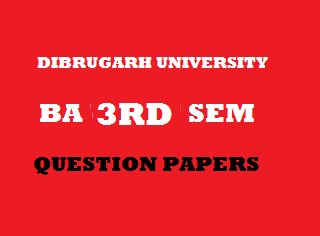## Wednesday, January 02, 20192012
(November)
ECONOMICS
(Major)
Course: 301
(Microeconomics - II)
Full Marks: 80
Pass Marks: 32
Time: 3 hours
The figures in the margin indicate full marks for the questions

1. Choose the correct one/Answer the following: 1x8=8
1. In perfect competition, the perfect knowledge about the market implies
1. Consumers know prices.
2. Producers know costs.
3. Workers know wage rate.
4. All of the above.
1. The shut-down price is equal to
1. Average cost.
2. Marginal cost.
3. Average variable cost.
4. Marginal revenue.
1. Which of the following forms of market has only one buyer?
1. Monopoly.
2. Discriminating Monopoly.
3. Monopsony.
4. Duopoly.
1. Who among the following has introduced the concept of Quasi-rent?
1. Hawley.
2. Marshall.
3. Ricardo.
4. Keynes.
1. Mention one situation when price discrimination is socially desirable.
2. What factors give monopoly power to a firm in monopolistic competition?
3. Who developed Innovation Theory of Profit?
4. Mention the condition of general equilibrium in production.
2. Write short notes on any four of the following: (within 150 words each): 4x4=16
1. Sources of monopoly power.
2. Scarcity vs. differential rent.
4. Collective bargaining.
5. Breakeven point.
6. Bilateral monopoly.
3. (a) Discuss the equilibrium of a firm under perfect competition both in the short run and long run with the help of a suitable diagram. 11
Or
(b) Short-run supply for competitive industry satisfies the law of supply. Why? Also show how long-run supply curve is derived under increasing cost industries. 5+6=11
4. (a) What are the necessary conditions of price discrimination? Show how a profit maximizing discriminating monopolist allocates his output between two markets and charges different prices. 4+8=12
Or
(b) Distinguish between a Monopoly and a Monopsony market. Explain long-run equilibrium of a monopoly firm. 4+8=12
5. (a) Explain, with the help of a diagram, price and output determination in a monopolistic competitive market.   11
Or
(b) Define oligopoly. What are its characteristics? Briefly explain different types of oligopoly.
2+4+5=11
6. (a) Distinguish between MRPL and VMPL. How is wage rate determined under the condition of monopolist in the product market and perfect competition in the labour market? 2+9=11
Or
(b) Explain critically Keynes Theory of Interest. 11
7. (a) What is general equilibrium? Explain general equilibrium of an exchange economy. 3+8=11
Or
(b) What is meant by interrelations and interdependence of market? Illustrate how a change in one market affects the equilibrium of another related market. 5+6=11

***